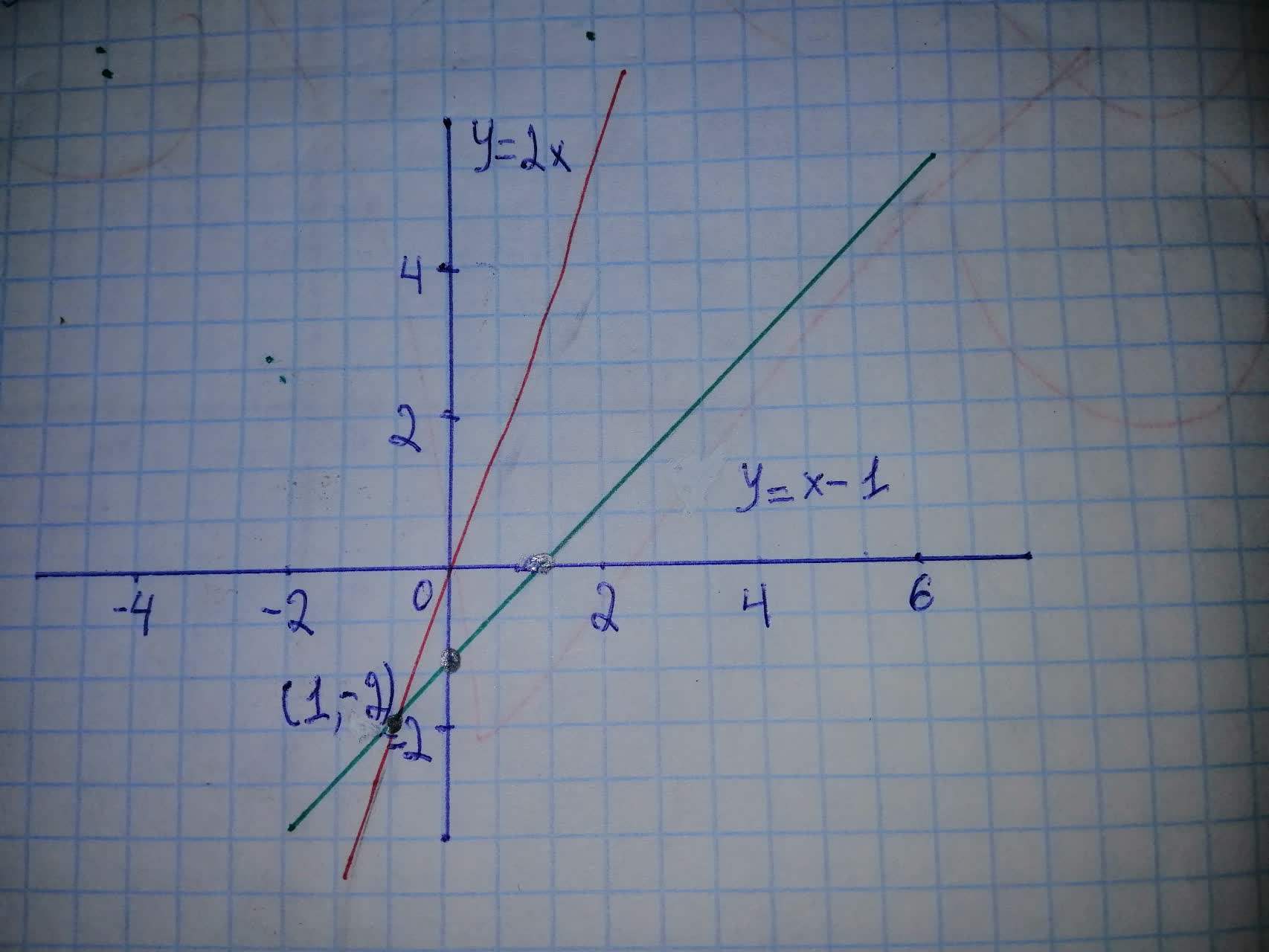# Solvin system of equations graphicallyvazelinahS 2020-10-27 Answered
Solvin system of equations graphically
You can still ask an expert for help

• Questions are typically answered in as fast as 30 minutes

Solve your problem for the price of one coffee

• Math expert for every subject
• Pay only if we can solve itun4t5o4v
Step 1
Take one example to understand the solution of systems by graphically.
Step 2
Now assume that and graph the equations in coordinate plane.Step 3 So using above graph we can say that (-1, -2) is the solution of